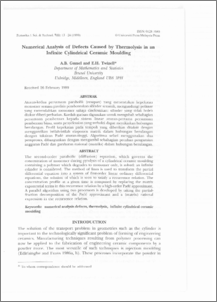# Numerical Analysis of Defects Caused by Thermolysis in an Infinite Cylindrical Ceramic Moulding

## Citation

Gumel, A. B. and Twizell, E. H. (1999) Numerical Analysis of Defects Caused by Thermolysis in an Infinite Cylindrical Ceramic Moulding. Pertanika Journal of Science & Technology, 7 (1). pp. 13-24. ISSN 0128-7680

## Abstract

The second-order parabolic (diffusion) equation, which governs the concentration of monomer during pyrolysis of a cylindrical ceramic moulding containing a polymer which degrades to monomer only, is solved: an infinite cylinder is considered. The method of lines is used to transform the partial differential equation into a system of first-order linear ordinary differential equations, the solution of which is seen to satisfy a recurrence relation. The concentration profile at a given time is computed by replacing the matrix exponential terms in this recurrence relation by a high-order Pade approximate. A parallel algorithm using two processors is developed by taking the partial fraction decomposition of the Pade approximate and a (matrix) rational expression in the recurrence relation.Preview
PDF
Numerical_Analysis_of_Defects_Caused_by_Thermolysis_in_an.pdfView Item